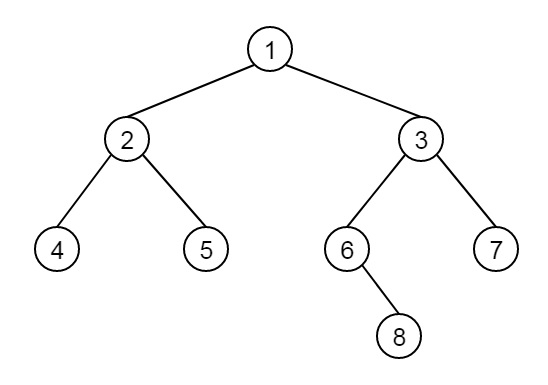# Find distance from root to given node in a binary tree in C++

Consider we have a binary tree with few nodes. We have to find the distance between the root and another node u. suppose the tree is like below:Now the distance between (root, 6) = 2, path length is 2, distance between (root, 8) = 3 etc.

To solve this problem, we will use a recursive approach to search the node at the left and right subtrees, and also update the lengths for each level.

## Example

Live Demo

#include<iostream>
using namespace std;
class Node {
public:
int data;
Node *left, *right;
};
Node* getNode(int data) {
Node* node = new Node;
node->data = data;
node->left = node->right = NULL;
return node;
}
int getDistance(Node *root, int x) {
if (root == NULL)
return -1;
int dist = -1;
if ((root->data == x) || (dist = getDistance(root->left, x)) >= 0 || (dist = getDistance(root->right, x)) >= 0)
return dist + 1;
return dist;
}
int main() {
Node* root = getNode(1);
root->left = getNode(2);
root->right = getNode(3);
root->left->left = getNode(4);
root->left->right = getNode(5);
root->right->left = getNode(6);
root->right->right = getNode(7);
root->right->left->right = getNode(8);
cout <<"Distance from root to node 6 is: " << getDistance(root,6);
cout << "\nDistance from root to node 8 is: " << getDistance(root,8);
}

## Output

Distance from root to node 6 is: 2
Distance from root to node 8 is: 3